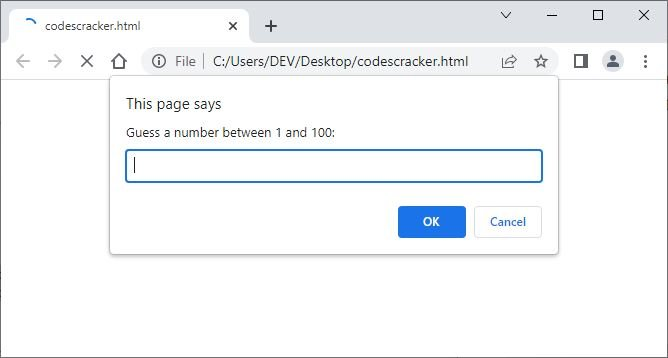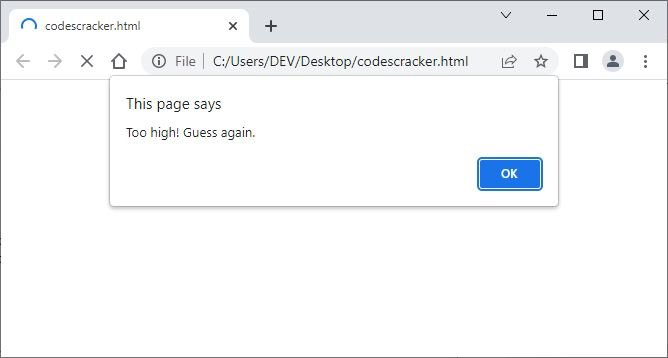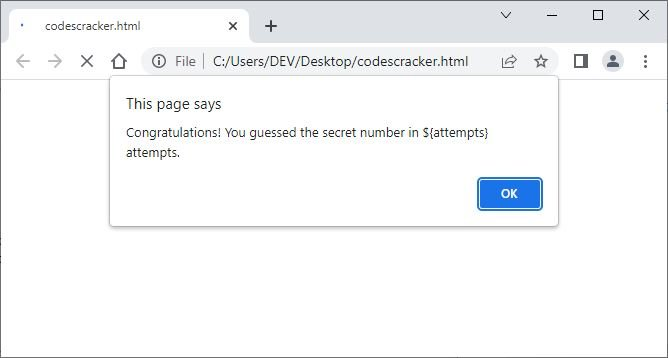# JavaScript keywords: definition, list, and examples

JavaScript keywords are reserved words that cannot be used as variable or function names due to their special significance in the language. For example:

HTML with JavaScript Code
```<!DOCTYPE html>
<html>
<body>

<p id="xyz"></p>

<script>
var x = 10;
if (x == 0) {
document.getElementById("xyz").innerHTML = "The value of 'x' is equal to 0.";
}
else if (x > 0) {
document.getElementById("xyz").innerHTML = "The value of 'x' is a positive number.";
}
else {
document.getElementById("xyz").innerHTML = "The value of 'x' is a negative number.";
}
</script>

</body>
</html>```
Output

In the above example, the var, if, and else are keywords. The var keyword is used to declare a variable. The if is used when we need to execute some block of code when the specified condition evaluates to be true. The else is used in conjunction with if to specify an alternative statement to execute when the if condition is false.

Note: Do not use any keyword as an identifier. If you do so, then that word acts the way it is defined by the developer. So avoid using any keyword as an identifier or variable.

An identifier is a name given to a variable, function, or object in JavaScript. It is used to identify and refer to a particular program element. Identifiers are case-sensitive and may contain letters, numbers, and underscores, but must begin with a letter, dollar sign, or underscore. Some valid identifiers in JavaScript are: myVariable, firstName, _myFunction, total_marks, \$myName, xyz, abc, etc.

## JavaScript keyword list

The following is a list of keywords available in the JavaScript language.

• await
• break
• case
• catch
• class
• const
• continue
• debugger
• default
• delete
• do
• else
• export
• extends
• false
• finally
• for
• function
• if
• implements
• import
• in
• instanceof
• interface
• let
• new
• null
• package
• private
• protected
• public
• return
• super
• switch
• static
• this
• throw
• true
• try
• typeof
• var
• void
• while
• with
• yield

Please be aware that certain keywords, such as "await" and "yield," are only used in asynchronous programming with generators and promises.

You will learn these keywords, or the ones that are most widely and frequently used, one by one as you progress through this JavaScript tutorial. But for now, I'm willing to write an example that uses and demonstrates the use of keywords in JavaScript:

```<script>
let secretNumber = Math.floor(Math.random() * 100) + 1;
let guess;
let attempts = 0;

while (guess !== secretNumber) {
guess = parseInt(prompt("Guess a number between 1 and 100:"));

if (isNaN(guess)) {
continue;
}

attempts++;

if (guess === secretNumber) {
alert("Congratulations! You guessed the secret number in \${attempts} attempts.");
} else if (guess < secretNumber) {
} else {
}
}

</script>```

Now, if you put the above JavaScript code in a file, say an HTML file called "codescracker.html," which makes it easy to execute directly through a web browser, the following is the initial output:That allows the user to guess a number between 1 and 100. So try to enter a number between 1 and 100 and hit the "OK" button to check if your guessed number is the same number that is generated randomly through the program at this particular execution. So if the guessed number does not match the randomly generated number, then the code will continue receiving or asking for the user to guess and enter the number.

If you enter a number, say 12, and hit the "OK" button, and if 12 is greater than the secretly or randomly generated number, then we will get an output like this:So because we get the message saying that the guessed number is less than 12, at our next attempt we need to try with a number less than 12. Therefore,  I tried 10 and I got the message "Too low," which makes me confirm that the number will be 11. So I tried 11, and here is the output I got.Now just click on the "OK" button to stop the execution of the code. That's it.

Now if we go through the demonstration of keywords used in the above JavaScript code, then I must say that there are multiple keywords, properties, and methods used. So here is the description of the keywords used in the above JavaScript code:

• let: used to declare and initialize variables (secretNumber, guess, and attempts).
• while: a loop statement that executes a block of code while a specified condition is true.
• if: a conditional statement that executes a block of code if a specified condition is true.
• continue: a keyword that stops the current iteration of a loop and continues with the next iteration.

Whatever the programming language we use, keywords are the terms that are required everywhere to define the particular task in the code, as described above. That is, when we need to declare a variable, we use let, var, or const. Or when we need to execute some block of code multiple times, we use while, for, or another keyword or statement, let's say do-while, and so on. So these are the usage and importance of keywords not only in JavaScript but also in other programming languages like "Java", "Python", "C" "C++", etc.

Keep in mind that other languages support different keywords with different syntax and formats.

JavaScript Online Test

« Previous Tutorial Next Tutorial »